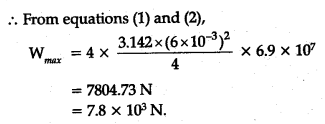# Two strips of metal are riveted together at their ends by four rivets, each of diameter 6 mm

Two strips of metal are riveted together at their ends by four rivets, each of diameter 6 mm. What is the maximum tension that can be exerted by the riveted strip if the shearing stress on the rivet is not to exceed 6.9 X 107 Pa ? Assume that each rivet is to carry one quarter of the load.

If the riveted strip is subjected to a stretching load W, the tensile force (i.e., tension) in each strip (equal to W) provides the shearing force on the four rivets. Since the load is shared uniformly, it means each
rivet is under a shearing force equal to W/4